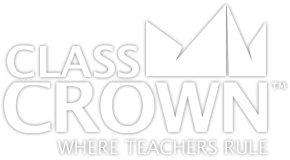# Number Lines: Repeated

## Number Line to 10: Repeated

Free printable, number line to 10 repeated for learning or teaching math skills on a number line.

## Number Line to 15: Repeated

Free printable, number line to 15 repeated for learning or teaching math skills on a number line.

## Number Line to 20: Repeated

Free printable, number line to 20 repeated for learning or teaching math skills on a number line.

## Number Line to 30: Repeated

Free printable, number line to 30 repeated for learning or teaching math skills on a number line.

## Number Line to 50: Repeated

Free printable, number line to 50 repeated for learning or teaching math skills on a number line.

## Number Line to 100: Repeated

Free printable, number line to 100 repeated for learning or teaching math skills on a number line.

## Number Line to 10: Blank, Repeated

Free printable, number line to 10 blank and repeated for learning or teaching math skills on a number line.

## Number Line to 15: Blank, Repeated

Free printable, number line to 15 blank and repeated for learning or teaching math skills on a number line.

## Number Line to 20: Blank, Repeated

Free printable, number line to 20 blank and repeated for learning or teaching math skills on a number line.

## Number Line to 30: Blank, Repeated

Free printable, number line to 30 blank and repeated for learning or teaching math skills on a number line.

## Number Line -10 to 10: Repeated

Free printable, number line negative 10 to 10 repeated for learning or teaching math skills on a number line.

## Number Line -15 to 15: Repeated

Free printable, number line negative 15 to 15 repeated for learning or teaching math skills on a number line.

## Number Line -20 to 20: Repeated

Free printable, number line negative 20 to 20 repeated for learning or teaching math skills on a number line.

## Number Line -30 to 30: Repeated

Free printable, number line negative 30 to 30 repeated for learning or teaching math skills on a number line.